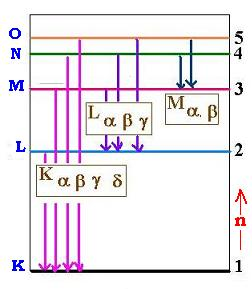Projectile's charge Z Projectile's E (MeV)

Contents

The nucleus

Statistical Mechanics

Related topics

# Moseley's law

### 1. Characteristic x rays

When an electron falls from L shell to K shell, the photon (electromagnetic radiation) produced is called the Kα ray. If the process is from M to K , the ray is Kβ and son on .. Electrons with lower values of n are more bounded to the nucleus than those with higher values.
The shells correponding to the stationary states are given letter names: the n = 1 shell is called K shell, n = 2, the L shell, and so on.
If enough energy is transferred to a K electron to dislodge it from the atom, the atom will be left with a vacancy in its K shell. The atom is most stable in its ground state, another electron from one of the higher shells will fill the inner-shell vacancy at lower energy emitting electromagentic radiation. We call this an x ray. This x ray carries the energy :
EK = E1 - E2,3, ...
This emitted x ray is known also as the characteristic x-rayFor each element, the energy levels are different, so the characteristic K x-rays are different.

### 2. Empirical Moseley's law

In 1913, H.G.J. Moseley, working in Rutherford's Manchester laboratory, was engaged in cataloguing the characteristic x-ray spectra of series of elements. He concentrated on the K shell and the L shell x rays produced in an x-rays tube.
By comparing frequencies, Moseley found the following empirical relationship, extracted from his plot Z versus the square root of the meaured frequency:
ν = (3cR/4)(Z - 1)2
Where : Z is the atomic number , c is the speed of light, and R is the Rydberg constant.

### 3. Moseley's law from Bohr's atom

Supposing that there are two electrons in the K shell. For an electron L shell, to fill the missing K shell electron, it feels an effective charge (Z - 1) (+ Ze due to the proton and - e due to the remainig K shell electron). Thus the transition between L and K shell (n = 2 → n = 1) involves a photon of wavelength :
1/λ = R (Z - 1)2 [1/12 - 1/22] = (3R/4)(Z - 1)2
Wich is the same formula found empirically above.Web ScientificSentence